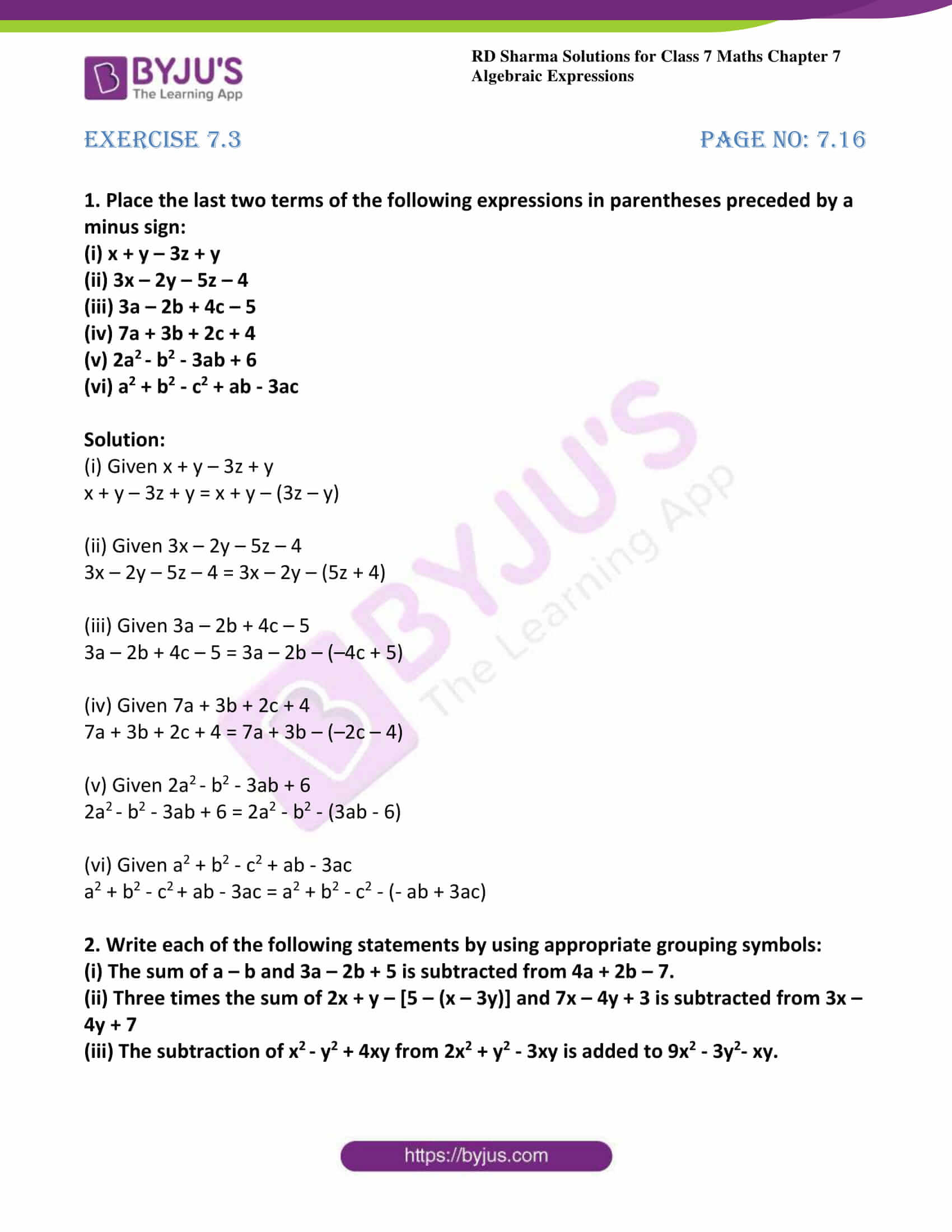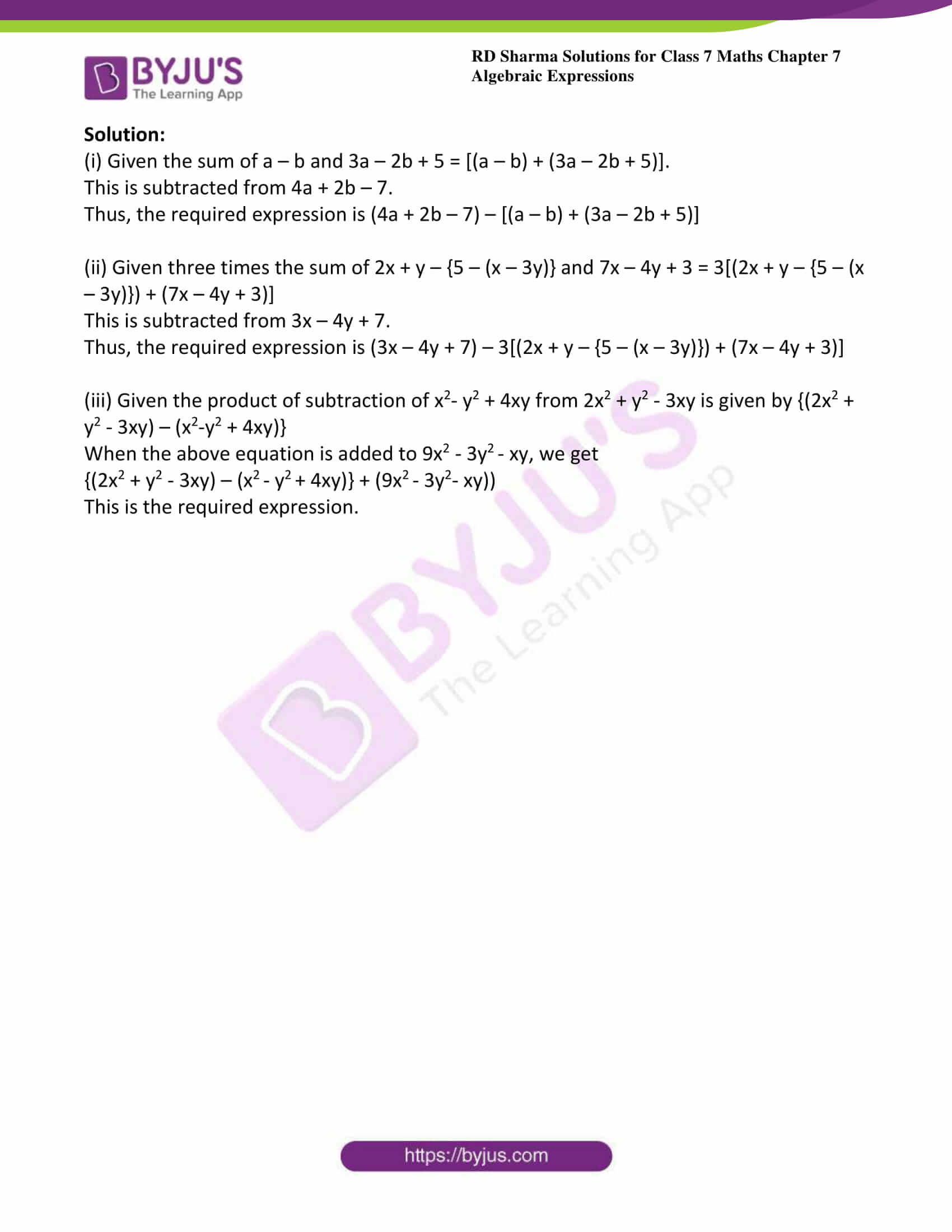# RD Sharma Solutions For Class 7 Maths Exercise 7.3 Chapter 7 Algebraic Expressions

RD Sharma Solutions for Class 7 Maths Exercise 7.3 of Chapter 7 Algebraic Expressions are provided here. The PDF of this exercise can be easily downloaded from the below links. BYJU’S subject matter experts have uniquely solved the exercise for your better understanding. By practising RD Sharma Solutions for Class 7, students can fulfil their wish by securing good marks in board exams. This exercise includes the use of grouping symbols or brackets in writing algebraic expressions. Already in Chapter 1, students learned about the removal of brackets, in the same way, they will perform with algebraic expressions.

## Download the PDF of RD Sharma Solutions For Class 7 Maths Chapter 7 – Algebraic Expressions Exercise 7.3### Access answers to Maths RD Sharma Solutions For Class 7 Chapter 7 – Algebraic Expressions Exercise 7.3

1. Place the last two terms of the following expressions in parentheses preceded by a minus sign:

(i) x + y – 3z + y

(ii) 3x – 2y – 5z – 4

(iii) 3a – 2b + 4c – 5

(iv) 7a + 3b + 2c + 4

(v) 2a– b2 – 3ab + 6

(vi) a2 + b2 – c2 + ab – 3ac

Solution:

(i) Given x + y – 3z + y

x + y – 3z + y = x + y – (3z – y)

(ii) Given 3x – 2y – 5z – 4

3x – 2y – 5z – 4 = 3x – 2y – (5z + 4)

(iii) Given 3a – 2b + 4c – 5

3a – 2b + 4c – 5 = 3a – 2b – (–4c + 5)

(iv) Given 7a + 3b + 2c + 4

7a + 3b + 2c + 4 = 7a + 3b – (–2c – 4)

(v) Given 2a– b2 – 3ab + 6

2a– b2 – 3ab + 6 = 2a2 – b2 – (3ab – 6)

(vi) Given a2 + b2 – c2 + ab – 3ac

a2 + b2 – c+ ab – 3ac = a2 + b2 – c2 – (- ab + 3ac)

2. Write each of the following statements by using appropriate grouping symbols:

(i) The sum of a – b and 3a – 2b + 5 is subtracted from 4a + 2b – 7.

(ii) Three times the sum of 2x + y – [5 – (x – 3y)] and 7x – 4y + 3 is subtracted from 3x – 4y + 7

(iii) The subtraction of x– y2 + 4xy from 2x2 + y2 – 3xy is added to 9x2 – 3y2– xy.

Solution:

(i) Given the sum of a – b and 3a – 2b + 5 = [(a – b) + (3a – 2b + 5)].

This is subtracted from 4a + 2b – 7.

Thus, the required expression is (4a + 2b – 7) – [(a – b) + (3a – 2b + 5)]

(ii) Given three times the sum of 2x + y – {5 – (x – 3y)} and 7x – 4y + 3 = 3[(2x + y – {5 – (x – 3y)}) + (7x – 4y + 3)]

This is subtracted from 3x – 4y + 7.

Thus, the required expression is (3x – 4y + 7) – 3[(2x + y – {5 – (x – 3y)}) + (7x – 4y + 3)]

(iii) Given the product of subtraction of x2– y2 + 4xy from 2x2 + y2 – 3xy is given by {(2x2 + y2 – 3xy) – (x2-y2 + 4xy)}

When the above equation is added to 9x2 – 3y– xy, we get

{(2x2 + y2 – 3xy) – (x– y+ 4xy)} + (9x– 3y2– xy))

This is the required expression.